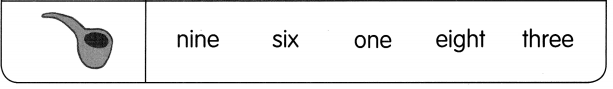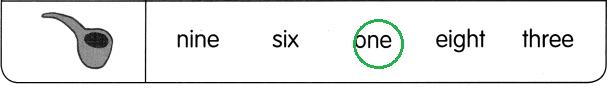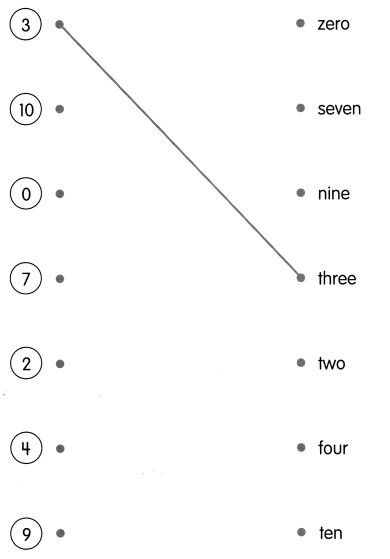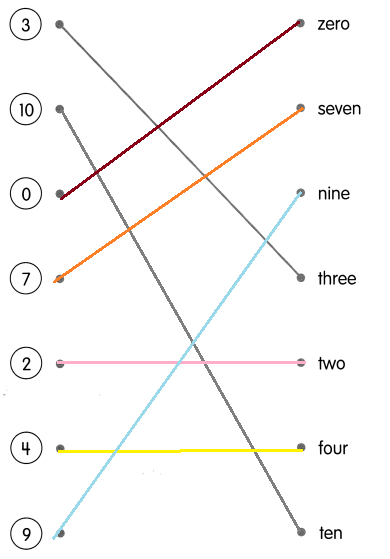# Math in Focus Grade 1 Chapter 1 Practice 1 Answer Key Counting to 10

This handy Math in Focus Grade 1 Workbook Answer Key Chapter 1 Practice 1 Counting to 10 detailed solutions for the textbook questions.

## Math in Focus Grade 1 Chapter 1 Practice 1 Answer Key Counting to 10

Count.

Write the numbers.

Example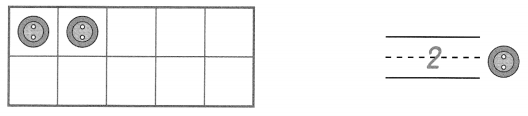Question 1.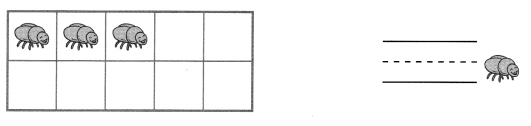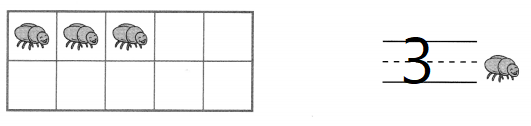Explanation:
Traced the number 3

Question 2.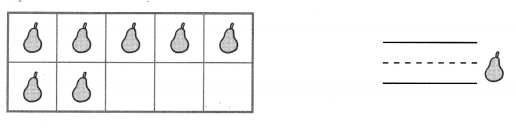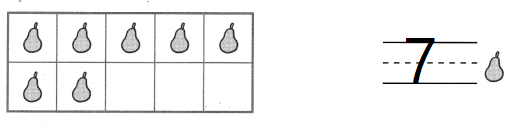Explanation:
There are 7 pears
traced the number 7

Question 3.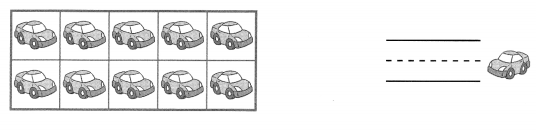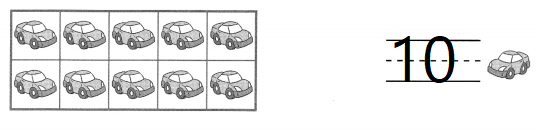Explanation:
There are 10 cars
traced the number 10

Count.
Write the numbers.

Question 4.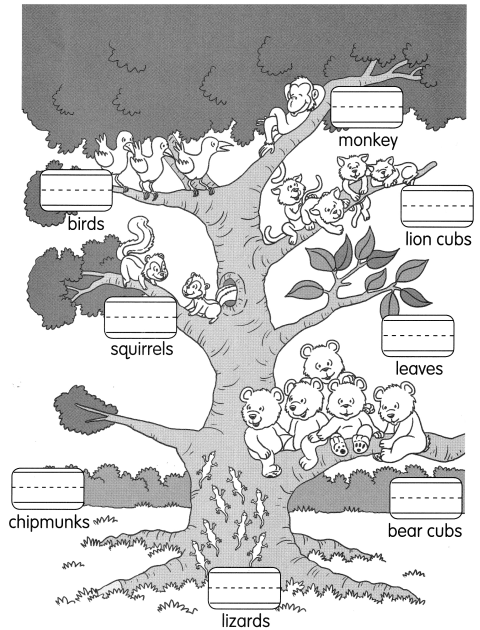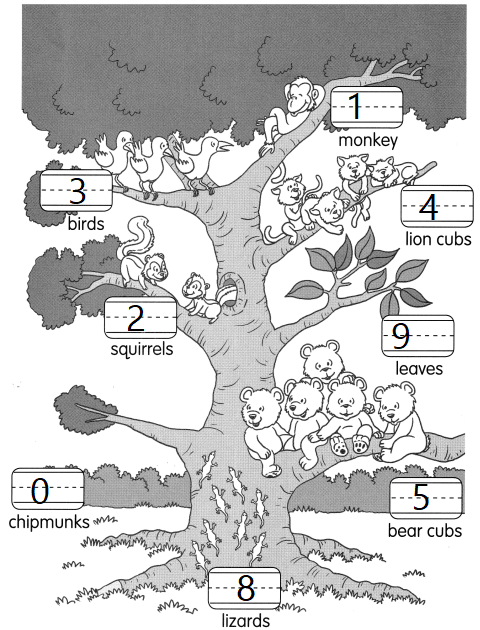Explanation:
Counted the animals on the tree
And traced the numbers

Draw.

Question 5.
A cow has 2 horns.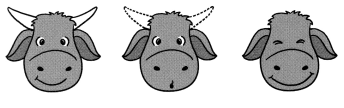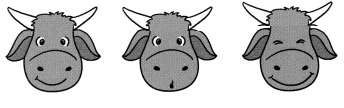Explanation:
Drawn the horns for remaining two cows
A cow has 2 horns.
and there are 3 cows

Question 6.
A chair has 4 legs.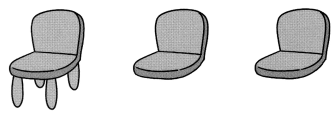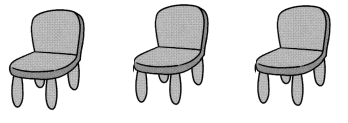Explanation:
Drawn the legs for remaining two chairs
A chair has 4 legs.
And there are 3 chairs.

Question 7.
An ant has 6 legs.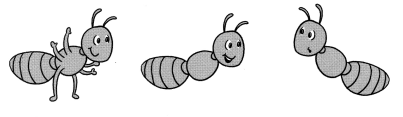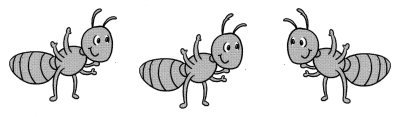Explanation:
Drawn the legs for remaining two ants
An ant has 6 legs.
And there are 3 ants.

Question 8.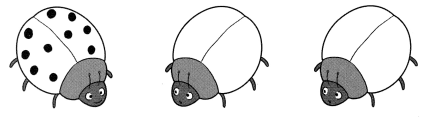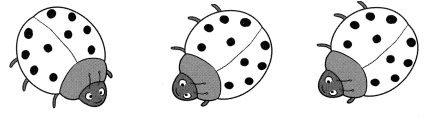Explanation:
Drawn the ten dots for remaining two bugs
There are 3 bugs.

How many insects are there?
Match.

Question 9.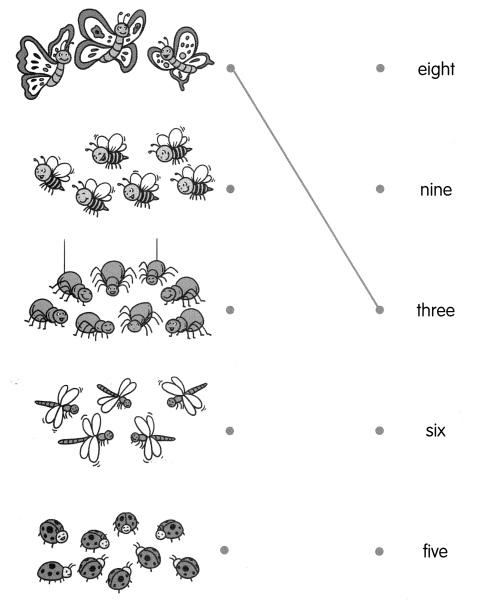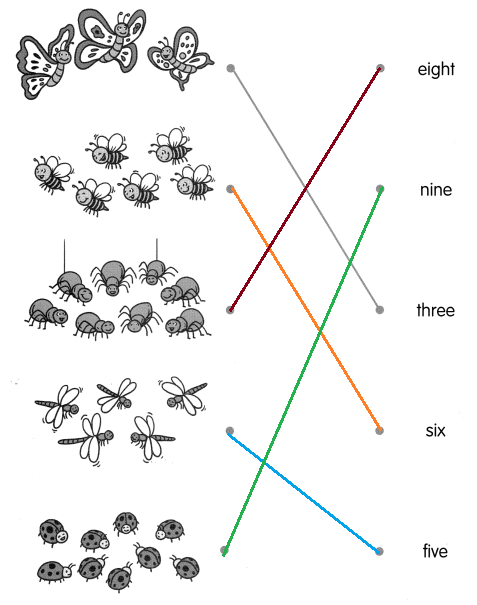Explanation:
There are 3 butterflies and matched the butterflies with the number 3
There are 6 honey bees and matched them with number 6
there are 8 spiders matched them with number 8
There are 5 wasp matched them with the number 5
there are 9 bugs matched them with the number 9

Count the things on the snowman. Circle the correct words.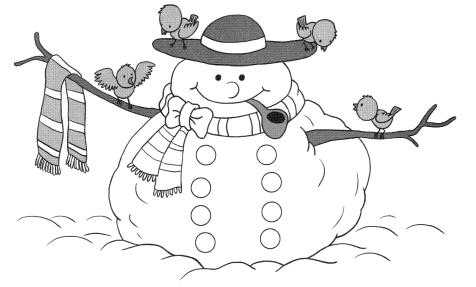Question 10.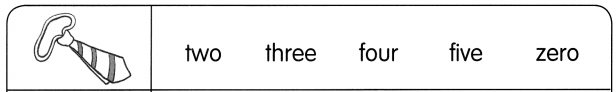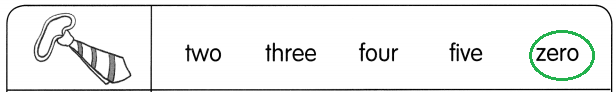Explanation:
There are no ties for the snow man
So, circled the zero

Question 11.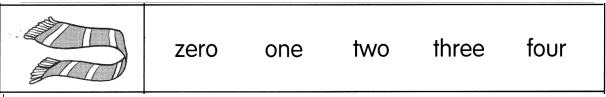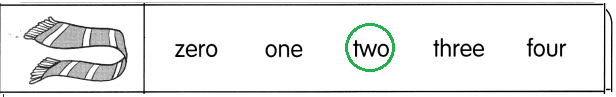Explanation:
There are 2 scarfs for the snow man
so, circled the number two

Question 12.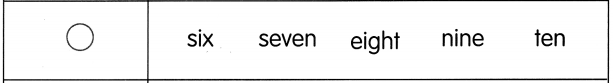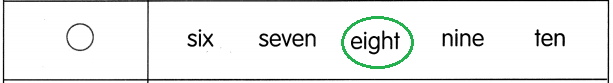Explanation:
There are 8 circles on the snow man dress
so, circled the number eight.

Question 13.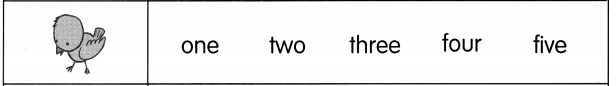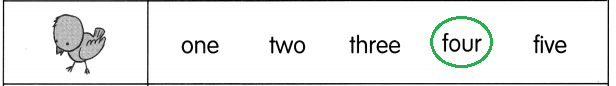Explanation:
There are four birds sitting on the tree in the picture of snow man
so, circled the number four.

Question 14.